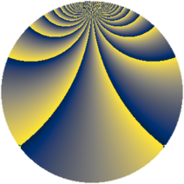# Properties

 Label 576.2.bdLevel $576$ Weight $2$ Character orbit 576.bd Rep. character $\chi_{576}(37,\cdot)$ Character field $\Q(\zeta_{16})$ Dimension $312$ Newform subspaces $3$ Sturm bound $192$ Trace bound $22$

# Related objects

## Defining parameters

 Level: $$N$$ $$=$$ $$576 = 2^{6} \cdot 3^{2}$$ Weight: $$k$$ $$=$$ $$2$$ Character orbit: $$[\chi]$$ $$=$$ 576.bd (of order $$16$$ and degree $$8$$) Character conductor: $$\operatorname{cond}(\chi)$$ $$=$$ $$64$$ Character field: $$\Q(\zeta_{16})$$ Newform subspaces: $$3$$ Sturm bound: $$192$$ Trace bound: $$22$$ Distinguishing $$T_p$$: $$5$$

## Dimensions

The following table gives the dimensions of various subspaces of $$M_{2}(576, [\chi])$$.

Total New Old
Modular forms 800 328 472
Cusp forms 736 312 424
Eisenstein series 64 16 48

## Trace form

 $$312q + 8q^{2} - 8q^{4} + 8q^{5} - 8q^{7} + 8q^{8} + O(q^{10})$$ $$312q + 8q^{2} - 8q^{4} + 8q^{5} - 8q^{7} + 8q^{8} - 8q^{10} + 8q^{11} - 8q^{13} + 8q^{14} - 8q^{16} + 8q^{17} - 8q^{19} + 8q^{20} + 8q^{23} - 8q^{25} + 48q^{26} + 32q^{28} + 8q^{29} + 48q^{32} + 32q^{34} + 8q^{35} - 8q^{37} + 48q^{38} + 32q^{40} + 8q^{41} - 8q^{43} + 16q^{44} - 8q^{46} + 8q^{47} - 8q^{49} - 16q^{50} + 40q^{52} + 8q^{53} - 72q^{55} - 48q^{56} - 80q^{58} + 72q^{59} - 8q^{61} - 56q^{62} + 88q^{64} + 16q^{65} + 8q^{67} - 40q^{68} + 88q^{70} + 72q^{71} - 8q^{73} - 48q^{74} - 40q^{76} + 8q^{77} - 40q^{79} - 80q^{80} - 88q^{82} + 8q^{83} - 8q^{85} - 96q^{86} - 88q^{88} + 8q^{89} - 8q^{91} - 144q^{92} - 104q^{94} - 128q^{98} + O(q^{100})$$

## Decomposition of $$S_{2}^{\mathrm{new}}(576, [\chi])$$ into newform subspaces

Label Dim. $$A$$ Field CM Traces $q$-expansion
$$a_2$$ $$a_3$$ $$a_5$$ $$a_7$$
576.2.bd.a $$56$$ $$4.599$$ None $$8$$ $$0$$ $$8$$ $$-8$$
576.2.bd.b $$128$$ $$4.599$$ None $$0$$ $$0$$ $$0$$ $$0$$
576.2.bd.c $$128$$ $$4.599$$ None $$0$$ $$0$$ $$0$$ $$0$$

## Decomposition of $$S_{2}^{\mathrm{old}}(576, [\chi])$$ into lower level spaces

$$S_{2}^{\mathrm{old}}(576, [\chi]) \cong$$ $$S_{2}^{\mathrm{new}}(64, [\chi])$$$$^{\oplus 3}$$$$\oplus$$$$S_{2}^{\mathrm{new}}(192, [\chi])$$$$^{\oplus 2}$$